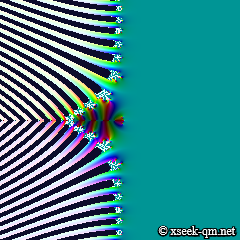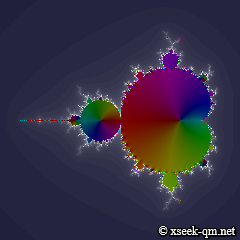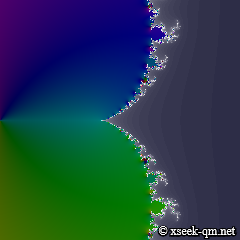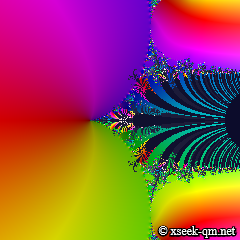# The Mandelbrot set of the zeta function

2013/9/28
Published 2013/7/21

I would like to show an animation of the Mandelbrot set of the zeta function. The interior of the Mandelbrot group is originally black, but here we are drawing the value of the complex number in color.

## The Mandelbrot set

The Mandelbrot set is a set that does not diverge to infinity in the following recurrence formula.

F (z ) = z 2
zk+1 = F (zk ) + c
z0 = 0

I modified the page to draw the Mandelbrot set of Mr. Kostas Symeonidis based on GPLv3 and made the following page by adding animation capabilities.

If you make a rectangle by dragging the mouse, you can expand its area. [Iterations] is the number of iterations of the calculation. Number of iterations of the initial state is 16. When you press the [Start] button to display the animation of the number of iterations increasing. You can observe the Mandelbrot Set is configured to correspond to the number of iterations increases.

When you expand the Mandelbrot set near the origin, you can see the following image.

We can see the spiral of the Mandelbrot set on the following page.

You will descend to the location of the image with the animation from the bird's eye view of the Mandelbrot set.

## The Mandelbrot set of exponential

The Mandelbrot set was configured with the following recurrence formula.

F (z ) = z 2
zk+1 = F (zk ) + c
z0 = 0

Is it possible to configure the set by the following recurrence formula of the exponential?

F (z ) = ez
zk+1 = F (zk ) + c
z0 = 0

We can configure the set by the above recurrence formula. We can confirm it on the following page.

Number of iterations of the initial state is 4. When you press the [Start] button to display the animation of the number of iterations increasing.

When you expand the Mandelbrot set near the origin, you can see the following image.

We can see the spiral of the Mandelbrot set of exponential on the following page. Please note that the page needs long calculation time.

## The zeta function

The zeta function is the function expressed by the following series.We can confirm the graph on the following page.

I used Canvas and Web Workers of the JavaScript to draw the screen. [Term number] is the number of terms of the calculating formula. Number of terms of the initial state is 16. When you press the [Start] button to display the animation of the number of terms increasing. You can observe the zeros are configured to correspond to the number of terms increases.

Description:

• It becomes larger in the order black, white, blue, light blue, sky-blue.
• A sequence of points on the left side of the screen is the trivial zeros.
• Black dots arranged in the vertical are the nontrivial zeros.
• White dot in the center is a pole

## The Mandelbrot set of the zeta function

The Mandelbrot set was configured with the following recurrence formula.

F (z ) = z 2
zk+1 = F (zk ) + c
z0 = 0

Is it possible to configure the set by the following recurrence formula of the zeta function?

F (z ) = ζ (z )
zk+1 = F (zk ) + c
z0 = 0

We can configure the set by the above recurrence formula. We can confirm it on the following page.The panoramic view of the Mandelbrot set of the zeta function

([Iterations]=4,[Term number]=16)

When you press the [Start] button of [Iterations] to display the animation of the number of iterations increasing. When you press the [Start] button of [Term number] to display the animation of the number of terms increasing.

When you expand the Mandelbrot set near the point of zero, you can see the following image. Please note that the page needs long calculation time.The Mandelbrot set of the zeta function near the point of zero

([Iterations]=64,[Term number]=8)

We can see the spiral of The Mandelbrot set of the zeta function on the following page. Please note that the page needs very long calculation time.The Mandelbrot set of the zeta function

([Iterations]=256,[Term number]=8)

The above picture looks like a flower.

## Comparison of the Mandelbrot sets

Each Mandelbrot set includes a similar image as shown in the following table.

Comparison of the Mandelbrot sets
F (z) z 2 ez ζ(z )
Panoramic
viewOrigin
/zeroSpiralI guess the following reasons.

• Exponential is expressed by the sum of a function of k-th power as shown below.• The zeta function is expressed by the sum of the exponential as shown below.## Physical meaning

What is the physical meaning of the set?

One point of the Mandelbrot set will converge or diverge to infinity. On the other hand, the universe retracts or expands endlessly. One point of the Mandelbrot set might correspond to a certain universe.

## The calculating formulas

The following calculating formula does not have good convergence.So we use the following calculating formulas.

• Asymptotic expansion
• Knopp-Hasse's calculating formula
• Peter Borwein's calculating formula

We confirm the arithmetic precision of each calculating formula on the following page.

I explain these formulas in the following sections.

### Asymptotic expansion of the zeta function

Euler introduced the asymptotic expansion of the zeta function by Euler-Maclaurin formula. When you press the button [ζ_AE] you can confirm it on the page of the graph.Here, Bk is the Bernoulli numbers. We use the Bernoulli polynomials Bk(1) as the Bernoulli numbers on this page. The variable q of the above formula is the number of terms ([Term number]). In addition, we calculate in r = q. We use the following formula in the domain with the number of terms more of 14 and more and the real part of s of -10 and less because a calculation error is large.The above calculating formula is the functional equation that mathematician Riemann showed in his paper in 1859. It is called "Riemann functional equation."

### Knopp-Hasse's calculating formula

Knopp conjectured the following calculating formula around 1930. The formula was proved by Hasse in 1930. When you press the button [ζ_KH] you can confirm it in the page of the graph.We use the Riemann functional equation in the domain with the number of terms of 27 and more and the real part of s of -7 and less because a calculation error is large.

### Peter Borwein's calculating formula

Peter Borwein published the following calculating formula in 2000. When you press the button [ζ_PB] you can confirm it in the page of the graph.We use the Riemann functional equation in the domain with the number of terms of 32 and more and the real part of s of -7 and less because a calculation error is large.

## An operational method

### Basic operation

• If you make a rectangle by dragging the mouse, you can expand its area.
• If you press the button of [Iterations], you can change the number of iterations.
• If you press the button of [Term number], you can change the number of terms of the formula.
• If you press the [Reset] button to return to its initial size.

### Applied operation

• When you press the [Start] button to display the animation to increase gradually the number of iterations ([Iterations]) or the number of the terms ([Term number]).
• When you press the [Stop] button to stop the animation.

### Other operation

• If you press the [Zoom out] button, you can zoom out the image.
• If you press the button of [Sizes], you can change the screen size.
• If you press the [PNG] button, you can output to a PNG image file.
• If you press the [Animation] button, you can watch the animation to the current image.

### Compliant browsers

• IE10 or later
• Chrome 3 or later
• Firefox 3.5 or later
• Opera 10.6 or later
• Safari 4 or later (Windows)
• Safari 5 or later (iOS)
• Chrome 19 or later (iOS)
• Drawing in Firefox seems faster on Windows.
• Drawing in Safari seems faster on iOS.

Related Articles:

©2013, xseek-qm.net

Sponsored Link